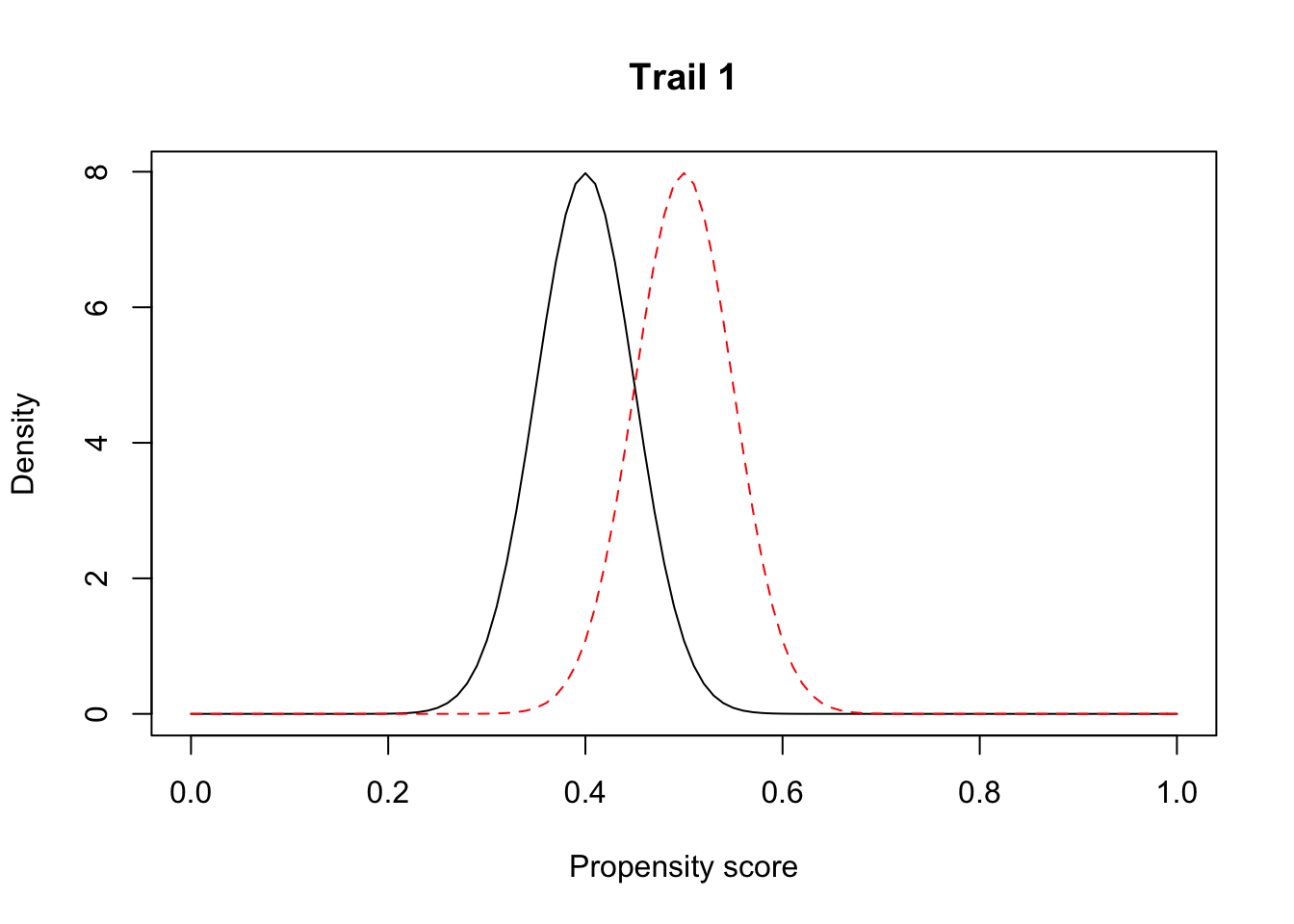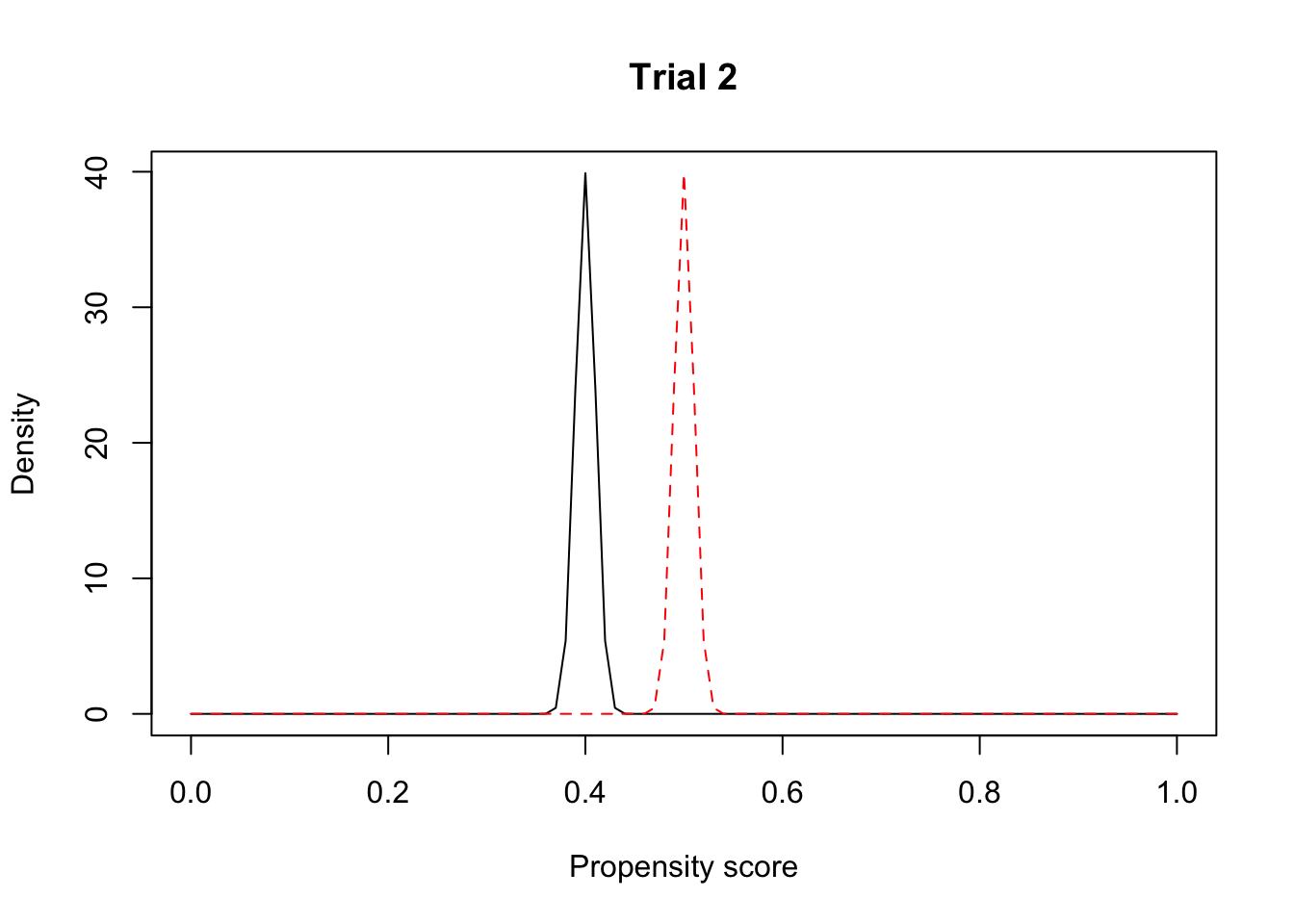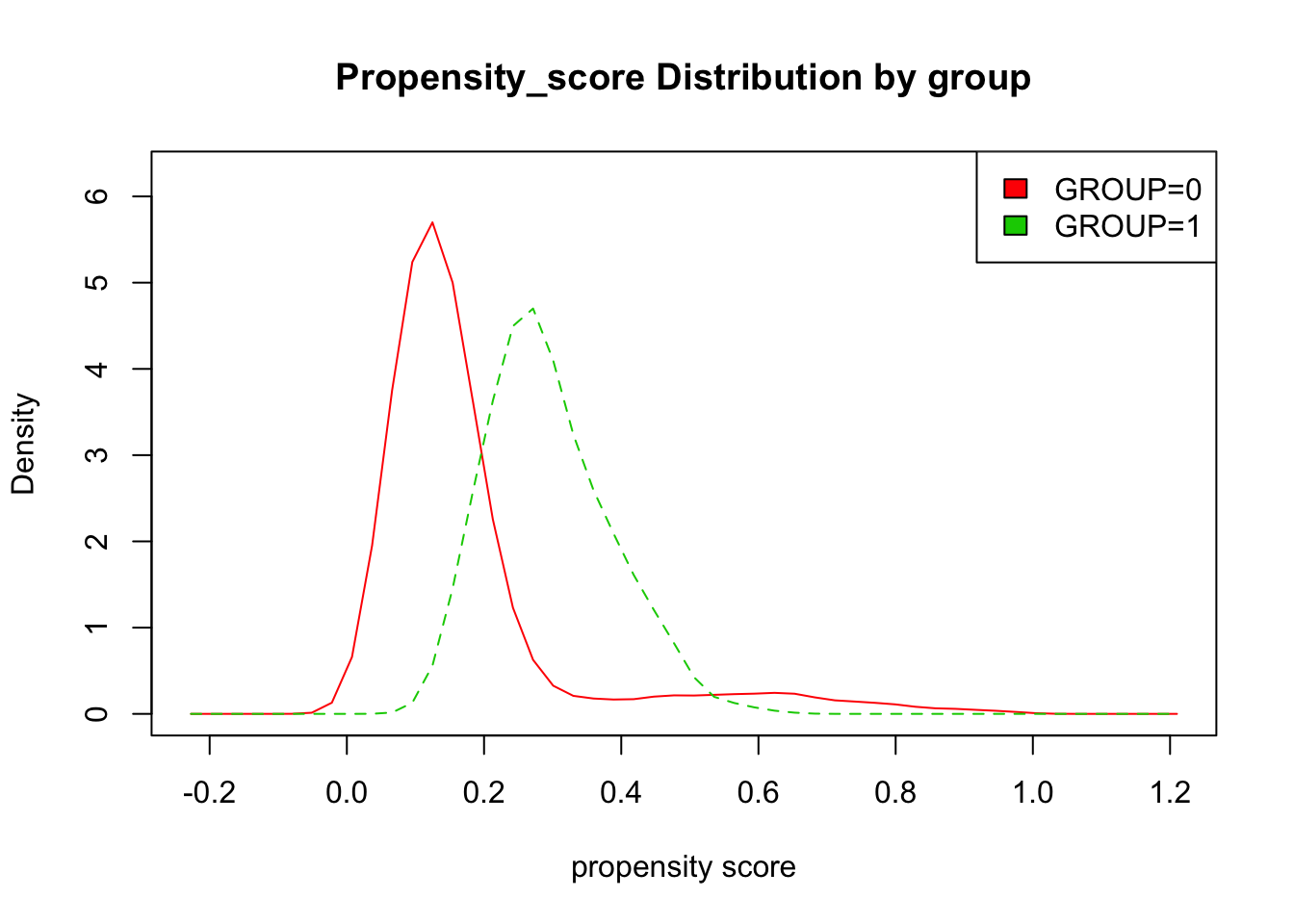# Tutorial: Propensity Score Weighting & Matching

## Propensity-score-based metrics

### Propensity-score-based method

In this section, we will introduce a propensity-score-based method to assess the generalizability of clinical study. We propose the use of propensity-score-based metrics to quantify the similarity of the participants in a designed clinical trial and a target population.

In Rubin Causal model, propensity score is defined as $$e(X)=Pr(Z=1|X)$$, where $$Z=z,z\in{0,1}$$ is an indicator variable denoting subject’s participation of trial, $$X = (X_1,X_2,…,X_n)$$ is a vector of covariates.

### Propensity-score difference

In [Elizabeth A. Stuart, 2010], the author introduces a propensity-score-based metric as:

$\Delta e(X) = \frac{1}{\sum I(Z_i=1)}\sum I(Z_i=1) e(X_i)-\frac{1}{\sum I(Z_i=0)}\sum I(Z_i=0)(1- e(X_i))$

However, we can find that this method has obvious shortcoming. Here we assume there are two trials. Two trials’ propensity score distributions are:

mu1 <- 0.4
mu2 <- 0.5
sigma1 <- 0.05
sigma2 <- 0.01

x <- seq(0,1,0.01)
f1 <- 1/sqrt(2*pi*sigma1^2)*exp(-(x-mu1)^2/(2*sigma1^2))
f2 <- 1/sqrt(2*pi*sigma1^2)*exp(-(x-mu2)^2/(2*sigma1^2))
f3 <- 1/sqrt(2*pi*sigma2^2)*exp(-(x-mu1)^2/(2*sigma2^2))
f4 <- 1/sqrt(2*pi*sigma2^2)*exp(-(x-mu2)^2/(2*sigma2^2))

matplot(x, cbind(f1,f2), type='l', main='Trail 1', xlab='Propensity score', ylab='Density', pch=1)matplot(x, cbind(f3,f4), type='l', main='Trial 2', xlab='Propensity score', ylab='Density', pch=2)If $$Y=1$$ means patients are eligible for the trial design while $$Y=0$$ means real world population. We find although the propensity score’s difference is same, which is $$0.5-0.4=0.1$$ here, variances of score distribution is different. We find the overlap of trial 2’ two distribution is larger than trial 1’s. We may wonder only using propensity score’s difference to describe the generalizability of trial is not enough.

#### Strictly standardized mean difference (SSMD) of propensity score

In [Ryoko Susukida,2016], the author uses SSMD to estimate the generalizability of trial. The definition of SSMD is $\frac{\Delta e(X)}{\text{pooled standard deviation}}$

And we find SSMD of trial 1 is $$\frac{0.5-0.4}{\sqrt{0.01}}=1$$, while SSMD of trial 2 is $$\frac{0.5-0.4}{\sqrt{0.05}}=0.447$$. According to [Ryoko Susukida,2016], propensity score mean values that differ by more than 0.25 standard deviations (standardized $$\Delta e(X)$$) indicate significant differences between the samples. The significant differences of trial 1 is higher than trial 2, which verify our former assumption.

### Run propensity-score-based method on R

We use a real world data to exhibit this propensity-score-based method

#### Introduction to Data Sets:

library(dplyr)
##
## Attaching package: 'dplyr'
## The following objects are masked from 'package:stats':
##
##     filter, lag
## The following objects are masked from 'package:base':
##
##     intersect, setdiff, setequal, union
T2D <- read.csv("NHANES_T2D_tutorial_10242019.csv",header=T)
T2D = mutate(T2D, GROUP = ifelse(T2D$BMXBMI<40 & T2D$LBXGH>7.5 & T2D$LBXGH<10 & T2D$RIDAGEYR>18 & T2D$RIDAGEYR<90, 1, 0)) head(T2D) ## SEQN RIAGENDR RIDRETH1 LBXGH BMXBMI RIDAGEYR GROUP ## 1 21043 1 4 5.4 62.77 31 0 ## 2 21093 2 2 12.1 28.97 64 0 ## 3 21099 1 3 5.8 40.41 75 0 ## 4 21104 1 4 6.5 29.33 70 0 ## 5 21112 1 5 7.3 21.65 76 0 ## 6 21118 1 1 5.5 25.76 66 0 use logistics regression model to estimate propensity score: m_ps <- glm(GROUP ~ RIDAGEYR + LBXGH + BMXBMI, data = T2D, family = binomial()) ## construct the model summary(m_ps) ## ## Call: ## glm(formula = GROUP ~ RIDAGEYR + LBXGH + BMXBMI, family = binomial(), ## data = T2D) ## ## Deviance Residuals: ## Min 1Q Median 3Q Max ## -2.6542 -0.6091 -0.4971 -0.3405 2.0400 ## ## Coefficients: ## Estimate Std. Error z value Pr(>|z|) ## (Intercept) -4.090327 0.516348 -7.922 2.34e-15 *** ## RIDAGEYR 0.012122 0.004429 2.737 0.0062 ** ## LBXGH 0.462886 0.029639 15.618 < 2e-16 *** ## BMXBMI -0.050434 0.008365 -6.029 1.65e-09 *** ## --- ## Signif. codes: 0 '***' 0.001 '**' 0.01 '*' 0.05 '.' 0.1 ' ' 1 ## ## (Dispersion parameter for binomial family taken to be 1) ## ## Null deviance: 2713.7 on 2694 degrees of freedom ## Residual deviance: 2371.4 on 2691 degrees of freedom ## AIC: 2379.4 ## ## Number of Fisher Scoring iterations: 5 prs_df <- data.frame(pr_score = predict(m_ps, type = "response"), GROUP = m_ps$model$GROUP) ## estimate the propensity score head(prs_df) ## show the head line of propensity score ## pr_score GROUP ## 1 0.01236198 0 ## 2 0.69536457 0 ## 3 0.07347589 0 ## 4 0.15287001 0 ## 5 0.29278493 0 ## 6 0.11470159 0 Now we want to compare the Strictly standardized mean difference (SSMD) of propensity scores of two groups. Here we will use the pooled standard deviation. Definition of pooled standard deviations: $\sigma=\sqrt{\frac{s1^2*(n_1-1)+s2^2*(n_2-1)}{(n_1+n_2-2)}}$ c_0 <- prs_df %>% filter(GROUP=="0") %>% select(pr_score) pr_score0 <- c_0$pr_score
c_1 <- prs_df %>%
filter(GROUP=="1") %>%
select(pr_score)
pr_score1 <- c_1$pr_score n0 = length(pr_score0) n1 = length(pr_score1) pooled_sd <- sqrt((sd(pr_score1)^2*(n1-1)+sd(pr_score0)^2*(n0-1))/(n0+n1-2)) prs_df %>% mutate(pooled_sd) %>% group_by(GROUP) %>% summarise(prs_mean = mean(pr_score), pooled_standard_deviations = mean(pooled_sd), standardized_prs_mean = mean(pr_score/pooled_sd), n = n()) ## # A tibble: 2 x 5 ## GROUP prs_mean pooled_standard_deviations standardized_prs_mean n ## <dbl> <dbl> <dbl> <dbl> <int> ## 1 0 0.179 0.145 1.24 2150 ## 2 1 0.294 0.145 2.04 545 prs_dis <- c(mean(pr_score1)-mean(pr_score0),pooled_sd,(mean(pr_score1)-mean(pr_score0))/pooled_sd) names(prs_dis) <- c("prs_difference","pooled_standard_deviations","standardized_prs_difference") data.frame(prs_dis) ## prs_dis ## prs_difference 0.1156551 ## pooled_standard_deviations 0.1445925 ## standardized_prs_difference 0.7998697 Compare propensity distributions for group with library(sm) ## Package 'sm', version 2.2-5.6: type help(sm) for summary information # create value labels GROUP.f <- factor(prs_df$GROUP, levels= c(0,1),
labels = c("GROUP=0","GROUP=1"))

# plot densities

sm.density.compare(prs_df$pr_score, prs_df$GROUP, xlab="propensity score")
title(main="Propensity_score Distribution by group")

# add legend via mouse click
colfill<-c(2:(2+length(levels(GROUP.f))))
legend("topright",legend = levels(GROUP.f), fill=colfill)